# Effect of batch size on training dynamics

This is a longer blogpost where I discuss results of experiments I ran myself.

In this experiment, I investigate the effect of batch size on training dynamics. The metric we will focus on is the generalization gap which is defined as the difference between the train-time value and test-time value of a metric you care about. We will investigate batch size in the context of image classification. Specifically, we will use the MNIST dataset. In our case, the generalization gap is simply the difference between the classification accuracy at test time and train time. These experiments were meant to provide some basic intuition on the effects of batch size. It is well known in the machine learning community the difficulty of making general statements about the effects of hyperparameters as behavior often varies from dataset to dataset and model to model. Therefore, the conclusions we make can only serve as signposts rather than general statements about batch size.

Some relevant papers on the topic of the batch size and generalization gaps include (some of my experiments reproduce the results in these papers):

Batch size is one of the most important hyperparameters to tune in modern deep learning systems. Practitioners often want to use a larger batch size to train their model as it allows computational speedups from the parallelism of GPUs. However, it is well known that too large of a batch size will lead to poor generalization (although currently it’s not known why this is so). For convex functions that we are trying to optimize, there is an inherent tug-of-war between the benefits of smaller and bigger batch sizes. On the one extreme, using a batch equal to the entire dataset guarantees convergence to the global optima of the objective function. However, this is at the cost of slower, empirical convergence to that optima. On the other hand, using smaller batch sizes have been empirically shown to have faster convergence to “good” solutions. This is intuitively explained by the fact that smaller batch sizes allow the model to “start learning before having to see all the data.” The downside of using a smaller batch size is that the model is not guaranteed to converge to the global optima. It will bounce around the global optima, staying outside some ϵ-ball of the optima where ϵ depends on the ratio of the batch size to the dataset size. Therefore, under no computational constraints, it is often advised that one starts at a small batch size, reaping the benefits of faster training dynamics, and steadily grows the batch size through training, also reaping the benefits of guaranteed convergence.

The picture is much more nuanced in non-convex optimization, which nowadays in deep learning refers to any neural network model. It has been empirically observed that smaller batch sizes not only has faster training dynamics but also generalization to the test dataset versus larger batch sizes. But this statement has its limits; we know a batch size of 1 usually works quite poorly. It is generally accepted that there is some “sweet spot” for batch size between 1 and the entire training dataset that will provide the best generalization. This “sweet spot” usually depends on the dataset and the model at question. The reason for better generalization is vaguely attributed to the existence to “noise” in small batch size training. Because neural network systems are extremely prone overfitting, the idea is that seeing many small batch size, each batch being a “noisy” representation of the entire dataset, will cause a sort of “tug-and-pull” dynamic. This “tug-and-pull” dynamic prevents the neural network from overfitting on the training set and hence performing badly on the test set.

In some ways, applying the analyse tools of mathematics to neural networks is analogous to trying to apply physics to the study of biological systems. Biological systems and neural networks, are much too complex to describe at the individual particle or neuron level. Often, the best we can do is to apply our tools of distribution statistics to learn about systems with many interacting entity. However, this almost always yields a coarse and incomplete understanding of the system at hand. This study is no exception.

# Problem statement

The precise problem that will be investigated is one of classification. Given an image X, the goal is to predict the label of the image y. In the case of the MNIST dataset, X are black-and-white images of the digits 0 to 9 and y are the corresponding digit labels “0” to “9”. Our model of choice is a neural network. Specifically, we will use a multi-layer perceptron (MLP) or informally “your average, vanilla neural network.”

Unless otherwise stated, the default model was used:

• 2 fully connected (FC) hidden layers, 1024 units each
• ReLU nonlinearities
• loss: negative log likelihood
• optimizer: SGD
• learning rate: 0.01
• epochs: 30

Ultimately the question we want to answer is “what batch size should I use when training a neural network?”

# Effect of batch size

For sanity sake, the first thing we ought to do to confirm the problem we are trying to investigate exists by showing the dependence between generalization gap and batch size. I’ve been referring to the metric we care about as the “generalization gap.” This is typically the measure of generalization authors use in papers but for simplicity in our study we only care about the test accuracy being as high as possible. As we will see, both the training and testing accuracy will depend on batch size so it’s more meaningful to talk about test accuracy rather than generalization gap. More specifically, we want the test accuracy after some large number of epochs of training or “asymptotic test accuracy” to be high. How many epochs is a “large number of epochs”? Ideally this is defined as the number of epochs of training required such that any further training provides little to no boost in test accuracy. In practice this is difficult to determine and we will have to make our best guess at how many epochs is appropriate to reach asymptotic behavior. I present the test accuracies of our neural network model trained using different batch sizes below.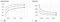Training loss and accuracy when the model is trained using different batch sizes.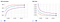Testing loss and accuracy when the model is trained using different batch sizes.
• Orange curves: batch size 64
• Blue curves: batch size 256
• Purple curves: batch size 1024

The x-axis shows the number of epochs of training. The y-axis is labelled for each plot. MNIST is obviously an easy dataset to train on; we can achieve 100% train and 98% test accuracy with just our base MLP model at batch size 64. Further, we see a clear trend between batch size and the asymptotic test (and train!) accuracy. We make our first conclusion: higher batch sizes leads to lower asymptotic test accuracy. This patterns seems to exist at its extreme for the MNIST dataset; I tried batch size equals 2 and achieved even better test accuracy of 99% (versus 98% for batch size 64)! As a forewarning, do not expect very low batch sizes such as 2 to work well in more complex datasets.

# Effect of Learning Rate

Some works in the optimization literature have shown that increasing the learning rate can compensate for larger batch sizes. With this in mind, we ramp up the learning rate for our model to see if we can recover the asymptotic test accuracy we lost by increasing the batch size.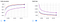Training loss and accuracy when the model is trained using different learning rates.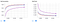Testing loss and accuracy when the model is trained using different learning rates.
• Orange curves: batch size 64, learning rate 0.01 (reference)
• Purple curves: batch size 1024, learning rate 0.01 (reference)
• Blue: batch size 1024, learning rate 0.1

The orange and purple curves are for reference and are copied from the previous set of figures. Like the purple curve, the blue curve trains with a large batch size of 1024. However, the blue cure has a 10 fold increased learning rate. Interestingly we can recover the lost test accuracy from a larger batch size by increasing the learning rate. Using a batch size of 64 (orange) achieves a test accuracy of 98% while using a batch size of 1024 only achieves about 96%. But by increasing the learning rate, using a batch size of 1024 also achieves test accuracy of 98%. Just as with our previous conclusion, take this conclusion with a grain of salt. It is known that simply increasing the learning rate does not fully compensate for large batch sizes in more complex datasets than MNIST.

# Can you recover good asymptotic behavior by lowering the batch size?

The next interesting question to ask is whether training with large batch sizes “starts you out on a bad path from which you can’t recover”. That is, if we start training with batch size 1024, then switch to batch size 64, can we still achieve the higher asymptotic test accuracy of 98%? I investigated three cases: train using a small batch size for a single epoch then switch to a large batch size, train using a small batch size for many epochs then switch to a larger batch size, and train using a large batch size then switch to a higher learning rate with the same batch size.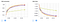Training loss and accuracy for different “recovery” scenarios.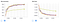Testing loss and accuracy for different “recovery” scenarios.
• Orange curves: train on batch size 64 for 30 epochs (reference)
• Neon yellow: train on batch size 1024 for 60 epochs (reference)
• Green curves: train on batch size 1024 for 1 epoch then switching to batch size 64 for 30 epochs (31 epochs total)
• Dark yellow curves: train on batch size 1024 for 30 epochs then switching to batch size 64 for 30 epochs (60 epochs total)
• Purple curves: training on batch size 1024 and increasing the learning rate 10 folds at epoch 31 (60 epochs total)

As before, the orange curves are for a small batch size. The neon yellow curves serve as a control to make sure we aren’t doing better on the test accuracy because we’re simply training more. If you pay careful attention to the x-axis, the epochs are enumerated from 0 to 30. This is because only the last 30 epochs of training are shown. For experiments with greater than 30 epochs of training in total, the first x − 30 epochs have been omitted.

It’s hard to see the other 3 lines because they’re overlapping but it turns out it doesn’t matter because all three cases we recover the 98% asymptotic test accuracy! In conclusion, starting with a large batch size doesn’t “get the model stuck” in some neighbourhood of bad local optimums. The model can switch to a lower batch size or higher learning rate anytime to achieve better test accuracy.

# Hypothesis: gradient competition

How do we explain why training with larger batch sizes leads to lower test accuracy? One hypothesis might be that the training samples in the same batch interfere (compete) with each others’ gradient. One sample wants to move the weights of the model in one direction while another sample wants to move the weights the opposite direction Therefore, their gradients tend to cancel and you get a small overall gradients. Perhaps if the samples are split into two batches, then competition is reduced as the model can find weights that will fit both samples well if done in sequence. In other words, sequential optimization of samples is easier than simultaneous optimization in complex, high dimensional parameter spaces.

The hypothesis is represented pictorially below. The purple arrow shows a single gradient descent step using a batch size of 2. The blue and red arrows show two successive gradient descent steps using a batch size of 1. The black arrow is the vector sum of the blue and red arrows and represents the overall progress the model makes in two steps of batch size 1. Both experiments start at the same weights in weight-space. While not explicitly shown in the image, the hypothesis is that the purple line is much shorter than the black line due to gradient competition. In other words, the gradient from a single large batch size step is smaller than the sum of gradients from many small batch size steps.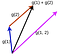Visualization of our gradient competition hypothesis.

The experiment involves replicating the picture shown above. We train the model to a certain state. Then the control group (purple arrow) is computed by finding the single-step gradient with batch size 1024. The experimental group (black arrow) is computed by making multiple gradient steps and finding the vector sum of those gradients using a smaller batch size. The product of the number of steps and batch size is fixed constant at 1024. This represents different models seeing a fixed number of samples. For example, for a batch size of 64 we do 1024/64=16 steps, summing the 16 gradients to find the overall training gradient. For batch size 1024, we do 1024/1024 = 1 step. Note that for the smaller batch sizes, different samples are drawn for each batch. The idea is to compare the gradients of the model for different batch sizes after the models have seen the same number of samples. As a last caveat, for simplicity we only measure the gradient for the last layer of our MLP model which has 1024 ⋅ 10 = 10240 weights.

We investigated the following batch sizes: 1, 2, 3 ,4 ,5, 6, 7, 8, 16, 32, 64, 128, 256, 512, 1024.

One trial meant:

1. Loading/resetting the model weights to a fixed trained point (I used the model weights after training for 2/30 epochs at 1024 batch size).
2. Randomly sampling 1024 data samples from the training set.
3. Step the model through all 1024 data samples once, with different batch sizes.

For each batch size, I repeated the experiment 1000 times. I didn’t take more data because storing the gradient tensors is actually very expensive (I kept the tensors of each trial to compute higher order statistics later on). The total size of the gradients tensor file was 600MB.

For each of the 1000 trials, I compute the Euclidean norm of the summed gradient tensor (black arrow in our picture). I then compute the mean and standard deviation of these norms across the 1000 trials. This is done for each batch size.

I wanted to investigate two different weight regimes: early on during training when the weights have not converged and a lot of learning is occurring and later on during training when the weights have almost converged and minimal learning is occurring. For the early regime, I trained the MLP model for 2 epochs with batch size 1024 and for the late regime, I trained the model for 30 epochs.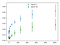Average euclidean norm of gradient tensors for different batch sizes at two regimes: early on during training and later during training.

The x-axis shows batch size. The y-axis shows the average Euclidean norm of gradient tensors across 1000 trials. The error bars indicate the variance of the Euclidean norm across 1000 trials. The blue points is the experiment conducted in the early regime where the model has been trained for 2 epochs. The green points is the late regime where the model has been trained for 30 epochs. As expected, the gradient is larger early on during training (blue points are higher than green points). Contrary to our hypothesis, the mean gradient norm increases with batch size! We expected the gradients to be smaller for larger batch size due to competition amongst data samples. Instead what we find is that larger batch sizes make larger gradient steps than smaller batch sizes for the same number of samples seen. Note that the Euclidean norm can be interpreted as the Euclidean distance between the new set of weights and starting set of weights. Therefore, training with large batch sizes tends to move further away from the starting weights after seeing a fixed number of samples than training with smaller batch sizes. The relation between batch size and gradient norm is √x. In other words, the relationship between batch size and the squared gradient norm is linear.

Furthermore, the variance is much lower for smaller batch sizes. However, what we might care about is the magnitude of the variance relative to the magnitude of the mean. Therefore, to make a more insightful comparison, I scale the both the mean and standard deviation of each batch size to the mean of batch size 1024. In other words,

where the bars represent normalized values and i denotes a certain batch size.

We’re justified in scaling mean and standard deviation of the gradient norm because doing so is equivalent to scaling the learning rate up for the experiment with smaller batch sizes. Essentially we want to know “for the same distance moved away from the initial weights, what is the variance in gradient norms for different batch sizes”? Keep in mind we’re measuring the variance in the gradient norms and not variance in the gradients themselves, which is a much finer metric.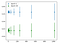Normalized average euclidean norm of gradient tensors.

We see a very surprising result above. For the same average Euclidean norm distance from the initial weights of the model, larger batch sizes have larger variance in the distance. That’s a mouthful! In short, given two models trained with different batch sizes, on any particular gradient step if the learning rate is adjusted such that both models move on average the same distance, the model with the larger batch size will vary more in how far it moves. This is somewhat counter-intuitive since it is well known that smaller batch sizes are “noisy” and therefore you might expect the variance of the gradient norm to be larger. Note that for each trial we are sampling 1024 different samples, rather than using the same 1024 samples across all trials. Also note that the variance between trials might be caused by two things: the different samples that are drawn from the dataset across different trials and the random seed for each trial (which wasn’t controlled but really should be). Moving forward, I’m going to guess the variance is caused by the first factor: different samples. This is an interesting result and two things remain to be done:

1. Characterize the functional relationship between batch size and the standard deviation of the average gradient norm.
2. Explain why the variance increases with batch size.

Here’s the same analysis but we view the distribution of the entire population of 1000 trials. Each trial for each batch size is scaled using μ_1024/μ_i as before. The vertical axis is the batch size from 2 to 1024. The horizontal axis is the gradient norm for a particular trial. Due to the normalization, the center (or more accurately the mean) of each histogram is the same. The purple plot is for the early regime and the blue plot is for the late regime.

We see the same conclusion as before: large batch size means the model makes very large gradient updates and very small gradient updates. The size of the update depends heavily on which particular samples are drawn from the dataset. On the other hand using small batch size means the model makes updates that are all about the same size. The size of the update only weakly depends on which particular samples that are drawn from the dataset.

This conclusion is valid whether the model is in the early or late regime of training.

For reference, here are the raw distributions of the gradient norms (same plots as previously but without the μ_1024/μ_i normalization).

Finally let’s plot the raw gradient values without computing the Euclidean norm. What I’ve done here is take each scalar gradient in the gradient tensor and put them into a bin on the real number line. We combine the weights from the tensors of all 1000 trials by sharing bins between trials. As before, the vertical axis represents the batch size. The horizontal axis represents the value of the gradient. Orange is the early regime and light blue is the late regime.

It’s hard to see, but at the particular value along the horizontal axis I’ve highlighted we see something interesting. Larger batch sizes has many more large gradient values (about 10⁵ for batch size 1024) than smaller batch sizes (about 10² for batch size 2). Note that the values have not been normalized by μ_1024/μ_i. In other words, the distribution of gradients for larger batch sizes has a much heavier tail. The number of large gradient values decreases monotonically with batch size. The center of the gradient distribution is quite similar for different batch sizes. In conclusion, the gradient norm is on average larger for large batch sizes because the gradient distribution is heavier tailed.

Next experiments: as I have hinted, it might be interesting to look at the functional relationship between batch size and the variance of the average gradient norm and provide theoretical justification for the relationship. Furthermore, we might want to analyze the direction of the gradients rather than simply looking at the magnitude. We still can’t explain “increasing the learning rate recovers asymptotic test accuracy.”

# Getting Far Enough

In the following experiment, I seek to answer why increasing the learning rate can compensate for larger batch sizes. We start with the hypothesis that larger batch sizes don’t generalize as well because the model cannot travel far enough in a reasonable number of training epochs.

The experimental setup is simple. I start by training our model for 30 epochs. I then computed the L_2 distance between the final weights and the initial weights. This experiment is designed under the assumption that maybe there are good optimas that are far away from the initial weights and certain training setups cannot reach these optimas; they have to settle for worse optimas closer to the initial weights.

The vertical axis represents different batch sizes (BS). The horizontal axis represents different learning rates (LR). Each cell shows the distance from the final weights to the initial weights (W), the distance from the final biases to the initial biases (B) and the test accuracy (A).

The best solutions seem to be about ~6 distance away from the initial weights and using a batch size of 1024 we simply cannot reach that distance. This is because in most implementations the loss and hence the gradient is averaged over the batch. This means for a fixed number of training epochs, larger batch sizes take fewer steps. However, by increasing the learning rate to 0.1, we take bigger steps and can reach the solutions that are farther away. In conclusion, better solutions can be far away from the initial weights and if the loss is averaged over the batch then large batch sizes simply do not allow the model to travel far enough to reach the better solutions for the same number of training epochs. Interestingly, in the previous experiment we showed that larger batch sizes move further after seeing the same number of samples.

To confirm our conclusion, I trained batch size 1024 using 0.01 learning rate for (1024/64) ⋅ 30 = 480 epochs. In this setup, the model takes the same number of total steps as it would if trained on batch size 64 for 30 epochs. Theoretically, this should allow the model to travel to the far away solutions. Under this training setup, we find:

W: 6.63, B: 0.11, A: 98%

Indeed the model is able to find the far away solution and achieve the better test accuracy.

Here is a plot of the distance from initial weights versus training epoch for batch size 64.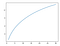Distance from initial weights versus training epoch number for SGD.

The x-axis represents the epoch number. The y-axis represents the distance from the initial weights. While the distance from initial weights increases monotonically over time, the rate of increase decreases. The plot for batch size 1024 is qualitatively the same and therefore now shown. However for the batch size 1024 case, the model tries to get far enough to find a good solution but doesn’t quite make it. All of these experiments suggest that for a fixed number of steps, the model is limited in how far it can travel using SGD, independent of batch size.

Closing remarks: so far we’ve found that the reason smaller batch sizes train more efficiently for the same number of epoch is because it takes more steps. For a given number of steps, it seems like there’s an upperbound on how far the model can travel away from its original weights. Therefore, smaller batch sizes means the model can find the faraway, better optima whereas large batch size means the model cannot. However, from my own experience working with more complex datasets this can’t be the whole story. I suspect that there are cases where the model travels just as far with a large batch size as a small batch size but still does worse than smaller batch size. Furthermore, we recall the train and test accuracy curves shown at the beginning of this study, they were both monotonically increasing. This is not representative of more complex datasets where the training accuracy usually increases monotonically while the test accuracy starts decreasing from overfitting. This could be an important difference for why the conclusions of this study do not generalize to more complex datasets.

# ADAM vs SGD

ADAM is one of the most popular, if not the most popular algorithm for researchers to prototype their algorithms with. Its claim to fame is insensitivity to weight initialization and initial learning rate choice. Therefore, it’s natural to extend our previous experiment to compare the training behavior of ADAM and SGD.

Recall that for SGD with batch size 64 the weight distance, bias distance, and test accuracy were 6.71, 0.11, and 98% respectively. Trained using ADAM with batch size 64, the weight distance, bias distance, and test accuracy are 254.3, 18.3 and 95% respectively. Note both models were trained using an initial learning rate of 0.01. ADAM finds solutions with much larger weights, which might explain why it has lower test accuracy and is not generalizing as well. This is why weight decay is recommended with ADAM.

Here is the distance from initial weights for ADAM.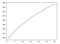Distance from initial weights versus training epoch number for ADAM.

This plot is almost linear whereas for SGD the plot was definitely sublinear. In other words, ADAM is less constrained to explore the solution space and therefore can find very faraway solutions.

For perspective let’s find the distance of the final weights to the origin.

SGD

• batch size 64, W: 44.9, B: 0.11, A: 98%
• batch size 1024: W: 44.1, B: 0.07, A: 95%
• batch size 1024 and 0.1 lr: W: 44.7, B: 0.10, A: 98%
• batch size 1024 and 480 epochs: W: 44.9, B: 0.11, A: 98%

ADAM

• batch size 64: W: 258, B: 18.3, A: 95%

We deduce that for SGD the weights are initialized to approximately the magnitude you want them to be (about 44) and most of the learning is shuffling the weights along the hyper-sphere of the initial radius (about 44). As for ADAM, the model completely ignores the initialization. Assuming the weights are also initialized with magnitude about 44, the weights travel to a final distance of 258.

Finally let’s plot the cosine similarity between the final and initial weights. We have 3 numbers, one for each of the 3 FC layers in our model.

SGD

• batch size 64, COS: [0.988 0.993 0.928]
• batch size 1024, COS: [0.998 0.998 0.978]
• batch size 1024 and 0.1 lr, COS: [ 0.991 0.994 0.936]
• batch size 1024 and 480 epochs, COS: [ 0.989 0.993 0.930]

ADAM

• batch size 64, COS: [ 0.124 0.359 0.153]

Again, we see that SGD leaves the initial weights almost untouched whereas ADAM completely ignores initialization. The second FC layer’s weights are appears to be the least changed (perhaps because the input and output layer learns to utilize the initialization of the second layer which shows the importance of a nonzero weight intialization: the model can just utilize the randomness of some layers instead of needing to learn that layer).

In summary we made the following findings in our experiments:

• higher batch sizes leads to lower asymptotic test accuracy
• we can recover the lost test accuracy from a larger batch size by increasing the learning rate
• starting with a large batch size doesn’t “get the model stuck” in some neighbourhood of bad local optimums. The model can switch to a lower batch size or higher learning rate anytime to achieve better test accuracy
• larger batch sizes make larger gradient steps than smaller batch sizes for the same number of samples seen
• for the same average Euclidean norm distance from the initial weights of the model, larger batch sizes have larger variance in the distance.
• large batch size means the model makes very large gradient updates and very small gradient updates. The size of the update depends heavily on which particular samples are drawn from the dataset. On the other hand using small batch size means the model makes updates that are all about the same size. The size of the update only weakly depends on which particular samples are drawn from the dataset
• the distribution of gradients for larger batch sizes has a much heavier tail
• better solutions can be far away from the initial weights and if the loss is averaged over the batch then large batch sizes simply do not allow the model to travel far enough to reach the better solutions for the same number of training epochs
• one can compensate for a larger batch size by increasing the learning rate or number of epochs so that the models can find faraway solutions
• for a fixed number of steps, the model is limited in how far it can travel using SGD, independent of batch size
• ADAM finds solutions with much larger weights, which might explain why it has lower test accuracy and is not generalizing as well
• for SGD the weights are initialized to approximately the magnitude you want them to be and most of the learning is shuffling the weights along the hyper-sphere of the initial radius. As for ADAM, the model completely ignores the initialization

As I mentioned at the start, training dynamics depends heavily on the dataset and model so these conclusions are signposts rather than the last word in understanding the effects of batch size.

## Mini Distill

This is a space where I write short summaries or extended…

## Mini Distill

This is a space where I write short summaries or extended tutorials of interesting papers I've read. The language is intended to be informal as to minimize the time between reading and understanding.

Written by

## Kevin Shen

MSc. at University of Toronto## Mini Distill

This is a space where I write short summaries or extended tutorials of interesting papers I've read. The language is intended to be informal as to minimize the time between reading and understanding.

## More From Medium

Medium is an open platform where 170 million readers come to find insightful and dynamic thinking. Here, expert and undiscovered voices alike dive into the heart of any topic and bring new ideas to the surface. Learn more

Follow the writers, publications, and topics that matter to you, and you’ll see them on your homepage and in your inbox. Explore

If you have a story to tell, knowledge to share, or a perspective to offer — welcome home. It’s easy and free to post your thinking on any topic. Write on Medium